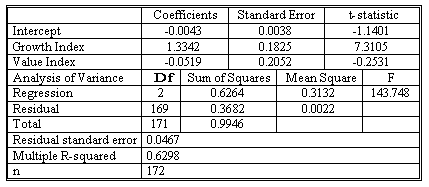### CFA Practice Question

There are 208 practice questions for this study session.

### CFA Practice Question

Suppose you want to know whether Fidelity Select Technology Fund (FSTF) behaves more like a large-cap growth fund or a large-cap value fund. You want to estimate the regression Y-hatt = b0 + b1X1t + b2X2t + et, where Yt is the monthly return to the FSTF, X1t is the monthly return to the S&P 500 Growth Index, and X2t is the monthly return to the S&P 500 Value index. The table below shows the results of a multiple linear regression using monthly data from December 1994 through March 2009.Use a 5% significance level and determine whether changes in the monthly return to the S&P 500 Value index affect FSTF's returns.
Correct Answer: The null hypothesis is H0: b2 = 0, and the alternative hypothesis is H1: b2 ≠ 0. The t-statistic is (-0.0519 - 0)/0.2052 = -0.2529. The t-test has 172 - 2 - 1 = 169 degrees of freedom. The critical value for the test statistic at the 0.025 significance level is about 1.96. As the absolute value of -0.2531 is less than 1.96, we fail to reject the null hypothesis that b2 = 0.